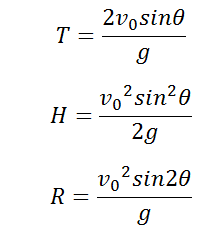# Parameters of a Parabolic Projectile on level field Calculator

This CalcTown calculator calculates the parameters of a parabolic projectile, like time of flight, maximum height and range when a body is projected from level ground.

m/s

#### Result

s
m
mClick here to view image

where

T=Time of Flight

R=Range of trajectory

H=Maximum Height reached by the point object

θ=Angle made by the projectile with the ground